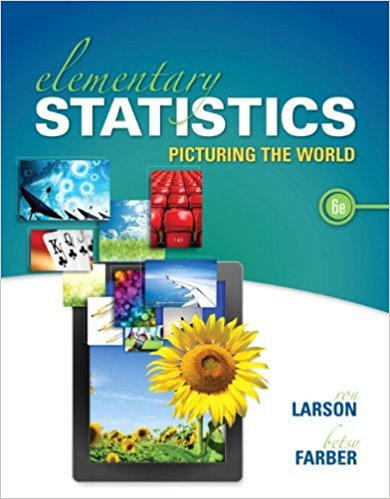×
×

# Solutions for Chapter 6: CONFIDENCE INTERVALS## Full solutions for Elementary Statistics: Picturing the World | 6th Edition

ISBN: 9780321911216Solutions for Chapter 6: CONFIDENCE INTERVALS

Solutions for Chapter 6
4 5 0 290 Reviews
31
2
##### ISBN: 9780321911216

This textbook survival guide was created for the textbook: Elementary Statistics: Picturing the World , edition: 6. Since 38 problems in chapter 6: CONFIDENCE INTERVALS have been answered, more than 99985 students have viewed full step-by-step solutions from this chapter. This expansive textbook survival guide covers the following chapters and their solutions. Elementary Statistics: Picturing the World was written by and is associated to the ISBN: 9780321911216. Chapter 6: CONFIDENCE INTERVALS includes 38 full step-by-step solutions.

Key Statistics Terms and definitions covered in this textbook
• a-error (or a-risk)

In hypothesis testing, an error incurred by failing to reject a null hypothesis when it is actually false (also called a type II error).

A formula used to determine the probability of the union of two (or more) events from the probabilities of the events and their intersection(s).

• All possible (subsets) regressions

A method of variable selection in regression that examines all possible subsets of the candidate regressor variables. Eficient computer algorithms have been developed for implementing all possible regressions

• Assignable cause

The portion of the variability in a set of observations that can be traced to speciic causes, such as operators, materials, or equipment. Also called a special cause.

• Average run length, or ARL

The average number of samples taken in a process monitoring or inspection scheme until the scheme signals that the process is operating at a level different from the level in which it began.

• Axioms of probability

A set of rules that probabilities deined on a sample space must follow. See Probability

• Consistent estimator

An estimator that converges in probability to the true value of the estimated parameter as the sample size increases.

• Correlation

In the most general usage, a measure of the interdependence among data. The concept may include more than two variables. The term is most commonly used in a narrow sense to express the relationship between quantitative variables or ranks.

• Counting techniques

Formulas used to determine the number of elements in sample spaces and events.

• Covariance

A measure of association between two random variables obtained as the expected value of the product of the two random variables around their means; that is, Cov(X Y, ) [( )( )] =? ? E X Y ? ? X Y .

• Covariance matrix

A square matrix that contains the variances and covariances among a set of random variables, say, X1 , X X 2 k , , … . The main diagonal elements of the matrix are the variances of the random variables and the off-diagonal elements are the covariances between Xi and Xj . Also called the variance-covariance matrix. When the random variables are standardized to have unit variances, the covariance matrix becomes the correlation matrix.

• Discrete uniform random variable

A discrete random variable with a inite range and constant probability mass function.

• Dispersion

The amount of variability exhibited by data

• Error variance

The variance of an error term or component in a model.

• Estimate (or point estimate)

The numerical value of a point estimator.

• Experiment

A series of tests in which changes are made to the system under study

• Fraction defective control chart

See P chart

• Generating function

A function that is used to determine properties of the probability distribution of a random variable. See Moment-generating function

• Geometric random variable

A discrete random variable that is the number of Bernoulli trials until a success occurs.

• Hat matrix.

In multiple regression, the matrix H XXX X = ( ) ? ? -1 . This a projection matrix that maps the vector of observed response values into a vector of itted values by yˆ = = X X X X y Hy ( ) ? ? ?1 .

×